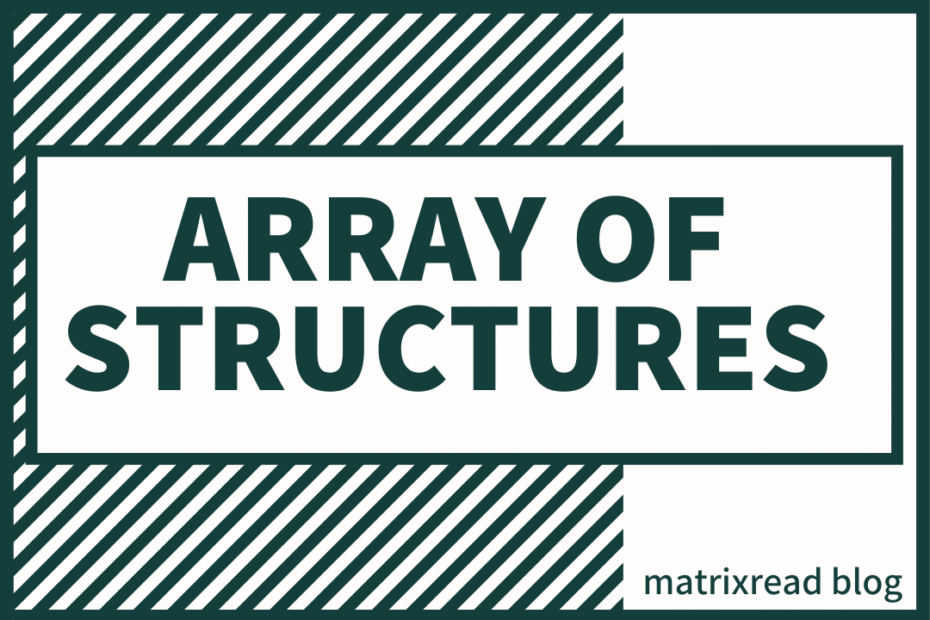# Array of Structures – API Problem

• DSA

In this post we’ll be solving a simple problem using an Array of Structures.

## Problem Statement:

Read an input of [N] API calls and find their Average, Each call has three timestamps [T1, T2, T3] and we need to give separate average for each.

Example

``````API.no  T1  T2  T3
1    2   3   2
2    5   3   1
1    2   1   2
2    1   1   1``````

#### Output

``````Api No: 1 T1:2 T2:2 T3:2
Api No: 2 T1:3 T2:2 T3:1``````

Explanation, in the above problem there are only two unique API numbers i.e 1 and 2. Each one of them are called two times so the average is (sum of timestamps)/2

## Solution Approach

Mind the duplicate calls, once we read an API call we need to do two things

1. If the same repeats again we need to add them to the previous Timestamps
2. Finally count the no.of same occurrences to calculate the average

## Array of Structures Code

``````#include <iostream>
using namespace std;

struct api
{
int n = 0, a = 0, b = 0, c = 0;
};

int main()
{
int n, i, s, a, b, c;
cin>>n;
struct api calls[n];
for (i = 1; i <=n; i++)
{
cin >> s >> a >> b >> c;
calls[s].a += a;
calls[s].b += b;
calls[s].c += c;
calls[s].n++;
}
for (i = 1; i <=n; i++)
{
if(calls[i].a+calls[i].b+calls[i].c==0)
break;
calls[i].a /= calls[i].n;
calls[i].b /= calls[i].n;
calls[i].c /= calls[i].n;
cout <<calls[i].a<<" "<<calls[i].b<<" "<<calls[i].c<<endl;
}
return 0;
}``````

## Reflecting

The structure is useful in this case, it made reading inputs and adding them easy. I had to use to loops because we can only calculate average when we finish reading all the inputs and we know the exact count of each API.no.

## Complexity

Time Complexity: O(N)

Time is linear here, Nothing complex.

Space Complexity: O(N)

We read the no.of inputs say 6, there are chances to get 2,4 or even 6 different API calls. Hence, we had to initialize all of them first and in the end a lot of space is left unused. For a problem using Array of Structures this is good but, a lot of space can be saved if the memory is allocated dynamically by using Linked List or something.

## Conclusion

Array of Structures is really cool, especially when we have inputs of different data types. And coming to the complexities, Time is linear nothing to fix and space can be decreased when allocated dynamically.